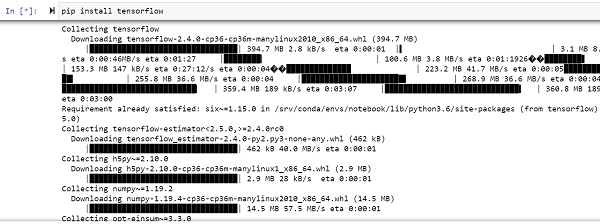# How can Tensorflow be used to sum specific elements/rows of a matrix in Python?

PythonServer Side ProgrammingProgramming

#### Beyond Basic Programming - Intermediate Python

Most Popular

36 Lectures 3 hours

#### Practical Machine Learning using Python

Best Seller

91 Lectures 23.5 hours

#### Practical Data Science using Python

22 Lectures 6 hours

Tensorflow is a machine learning framework that is provided by Google. It is an open−source framework used in conjunction with Python to implement algorithms, deep learning applications and much more. It is used in research and for production purposes.

It has optimization techniques that help in performing complicated mathematical operations quickly. This is because it uses NumPy and multi−dimensional arrays. These multi−dimensional arrays are also known as ‘tensors’. The framework supports working with deep neural network. It is highly scalable, and comes with many popular datasets. It uses GPU computation and automates the management of resources.

The ‘tensorflow’ package can be installed on Windows using the below line of code −

pip install tensorflow

Tensor is a data structure used in TensorFlow. It helps connect edges in a flow diagram. This flow diagram is known as the ‘Data flow graph’. Tensors are nothing but multidimensional array or a list.

We will be using the Jupyter Notebook to run these code. TensorFlow can be installed on Jupyter Notebook using ‘pip install tensorflow’.Following is an example −

## Example

import tensorflow as tf
import numpy as np

matrix_1 = tf.Variable([[1,2,3],[4,5,8],[9,10,0]])
print("The matrix is ")
print (matrix_1)

print("The sum of all elements ")
result = tf.reduce_sum(matrix_1)
print(result)
print("The sum of specific rows is")
result = tf.reduce_sum(matrix_1, 1)
print(result)

## Output

The matrix is
<tf.Variable 'Variable:0' shape=(3, 3) dtype=int32, numpy=
array([[ 1, 2, 3],
[ 4, 5, 8],
[ 9, 10, 0]], dtype=int32)>
The sum of all elements
tf.Tensor(42, shape=(), dtype=int32)
The sum of specific rows is
tf.Tensor([ 6 17 19], shape=(3,), dtype=int32)

## Explanation

• Import the required packages and provide an alias for it, for ease of use.

• A matrix is created using the Numpy package.

• The ‘reduce_sum’ function is used to find the sum of all values of the matrix.

• If a specific value is passed to the ‘reduce_sum’ apart from passing the matrix, it calculates the sum of every row.

• The resultant output is displayed on the console.